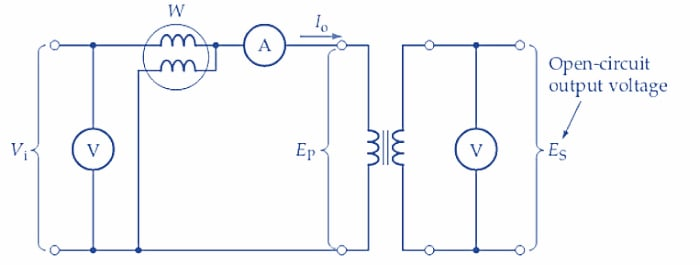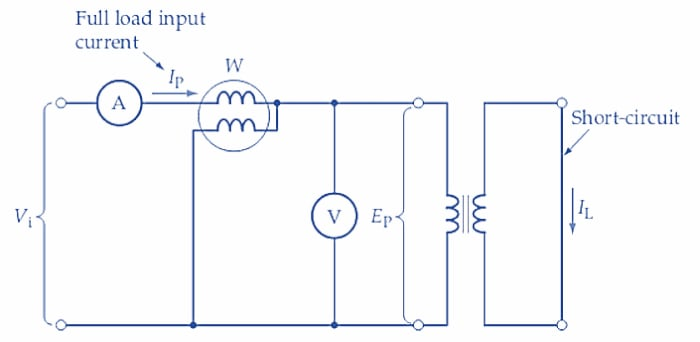Technical Article

# Open-Circuit and Short-Circuit Tests in Transformers

September 09, 2022 by Amna Ahmad

## A transformer could be tested under no-load and full-load conditions to determine its turns ratio, regulation, and efficiency. However, without fully loading the transformer, it is possible to perform two tests (open-circuit and short-circuit) from which all the important data can be derived. In this article, learn how to analyze the results of open-circuit and short-circuit transformer tests to determine the component values of the transformer equivalent circuit.

To discuss transformer theory accurately, we must take into account some losses associated with transformers. Generally, these losses can be divided into two categories: magnetic, or core, losses, and I2R, or coil loss. The core losses are relatively constant, resulting from the magnetic circuit that does not change much as transformer current changes. Currents cause the I2R, or coil loss, and because currents change, so do the coil losses.

All the losses associated with the core of the transformer are magnetic in nature and are relatively constant. The effect of hysteresis and eddy currents do not change much because of current flow; they result from core material and design.

A transformer is just like any electrical circuit or device. When current flows, it always creates a magnetic field and heat. The heat produced by the current flow in a transformer is referred to as the coil, or copper, loss. The formula for calculating this loss is the same as the formula for wattage: I2R.

The important thing to remember about coil loss is that the amount of coil loss is directly related to the current flow through the transformer and manifests itself as heat. To reduce this heat loss, transformer coils may be wound with copper conductors, which have less resistance than aluminum conductors of the same size.

Two tests (open-circuit and short-circuit) are performed to determine core and copper losses in the transformer. These losses are further used to compute the transformer’s efficiency.

$Efficiency=\frac{Output\,Power}{Input\,Power}\times100=\frac{Output\,Power}{Output+\Sigma(Losses)}\times100$

$Efficiency=\frac{Output\,Power}{Output\,Power+Core\,Losses+Copper\,Losses}\times100$

### Open-Circuit Test

Figure 1(a) shows the circuit for the transformer open-circuit test. The alternating input voltage is set to the normal primary level for the transformer, and the voltage at the open-circuited output terminals is monitored on a voltmeter, as illustrated. The wattmeter measures the input power, and the ammeter measures the primary current. Because the secondary is open-circuited, the primary current is very small, and the voltage drops across the ammeter and wattmeter can be assumed to be negligible. In this case, the input voltage can be taken as the transformer's primary voltage; thus, the ratio of the voltmeter readings gives the turns ratio.

$\frac{E_{P}}{E_{S}}=\frac{N_{P}}{N_{S}}$##### Figure 1(a). A transformer open-circuit test is performed by measuring the (no-load) secondary voltage, the primary current, and the input voltage and power. Image used courtesy of Amna Ahmad

With a very small primary current, and near-zero secondary current (i.e., the voltmeter current), the copper loss in the windings can be assumed negligible. The input power measured on the wattmeter is then the total transformer core losses, and the ammeter indicates the no-load primary current (Io) (see Figure 1(b)). From the measured values of input voltage, current, and power, the components of the no-load equivalent circuit can be determined.##### Figure 1(b). Secondary components referred to the primary. Image used courtesy of Amna Ahmad

True Power,

$P=\frac{E^{2}_{P}}{R_{o}}$

Or

$$R_{o}=\frac{E^{2}_{P}}{P}$$   (1)

Apparent Power,

$S=E_{P}I_{o}$

And

$S=\sqrt{(true\,power)^{2}+(reactive\,power)^{2}}=\sqrt{P^{2}+Q^{2}}$

Giving

$Q=\sqrt{S^{2}-P^{2}}$

Or

$$Q=\sqrt{(E_{P}I_{o})^{2}-P^{2}}$$   (2)

The reactive power can be determined by,

$Q=\frac{E^{2}_{P}}{X_{o}}$

So

$$X_{o}=\frac{E^{2}_{P}}{Q}$$   (3)

Example 1

An open-circuit test on a transformer produced the following measurements: EP=115 V, ES=57.5 V, P =9.5 W, and Io=180 mA. Determine the transformer turns ratio and the values of Ro and Xo.

Solution

$\frac{N_{S}}{N_{P}}=\frac{E_{S}}{E_{P}}=\frac{57.5V}{115V}=\frac{1}{2}$

From Equation 1,

$R_{o}=\frac{E^{2}_{P}}{P}=\frac{(115\,V)^{2}}{9.5\,W}=1.39k\Omega$

From Equation 2,

$Q=\sqrt{(E_{P}I_{o})^{2}-P^{2}}=\sqrt{(115V\times180mA)^{2}-(9.5W)^{2}}==18.39vars$

From Equation 3,

$X_{o}=\frac{E^{2}_{P}}{Q}=\frac{(115V)^{2}}{18.39\,vars}=719\Omega$

### Short-Circuit Test

The transformer short-circuit test is performed with the secondary terminals short-circuited, as illustrated in Figure 2(a). Note that the primary voltage (EP) is measured right at the transformer primary terminals to avoid error due to the voltage drops across the ammeter and wattmeter. The input voltage is increased from zero until the ammeter in the primary circuit indicates normal full-load primary current. When this occurs, the normal full-load secondary current is circulating in the secondary winding. Because the secondary terminals are short-circuited, the input voltage required to produce full-load primary and secondary currents is around 3% of the normal input voltage level. With such a low input voltage level, the core losses are so small that they can be neglected. However, the windings are carrying normal full-load current, and so the input is supplying the normal full-load copper losses.##### Figure 2(a). To perform a transformer short-circuit test, the secondary is shorted, and the primary current is adjusted to the normal full-load level. The primary voltage and input power are measured. Image used courtesy of Amna Ahmad

The output power (to the short-circuit) is zero, so the wattmeter measuring true input power indicates the full-load copper losses. The product of the ammeter and voltmeter readings gives the apparent input power. From these quantities, calculations may be made of the resistive and reactive components of the full-load equivalent circuit referred to the primary (see Figure 2(b)).##### Figure 2(b). Equivalent Transformer Circuit simplified by neglecting Ro and Xo. Image used courtesy of Amna Ahmad

True Power

$P=I^{2}_{P}R_{e}$

So

$$R_{e}=\frac{P}{I^{2}_{P}}$$   (4)

Apparent Power,

$S=E_{P}I_{P}$

Reactive Power,

$$Q=\sqrt{(E_{P}I_{P})^{2}-P^{2}}$$   (5)

The reactive power can be determined by,

$Q=I^{2}_{P}X_{e}$

So

$$X_{e}=\frac{Q}{I^{2}_{P}}$$   (6)

Example 2

Determine Re and Xe for the transformer in Example 1 when the following measurements were made on a short-circuit test: EP(SC) =5.5 V, IP=1 A, and P=5.25 W.

Solution

From Equation 4,

$R_{e}=\frac{P}{I^{2}_{P}}=\frac{5.25W}{(1A)^{2}}=5.25\Omega$

From Equation 5,

$Q=\sqrt{(E_{P}I_{P})^{2}-P^{2}}=\sqrt{(5.5V\times1A)^{2}}-(5.25W)^{2}=1.64\,var$

From Equation 6,

$X_{e}=\frac{Q}{I^{2}_{P}}=\frac{1.64\,var}{(1A)^{2}}=1.64\Omega$

### Key Takeaways of Open-Circuit and Short-Circuit Transformer Tests

The performance of a transformer is described in terms of its voltage regulation and efficiency, and can be predicted from the results of two tests: the open-circuit test and the short-circuit test. An open-circuit test is accomplished by measuring the (no-load) secondary voltage, the primary current, and the input voltage and power. In order to conduct a short-circuit test, the secondary side is shorted, and the primary current is adjusted to the normal full-load level. The primary voltage and input power are measured.

##### Featured image used courtesy of Adobe Stock